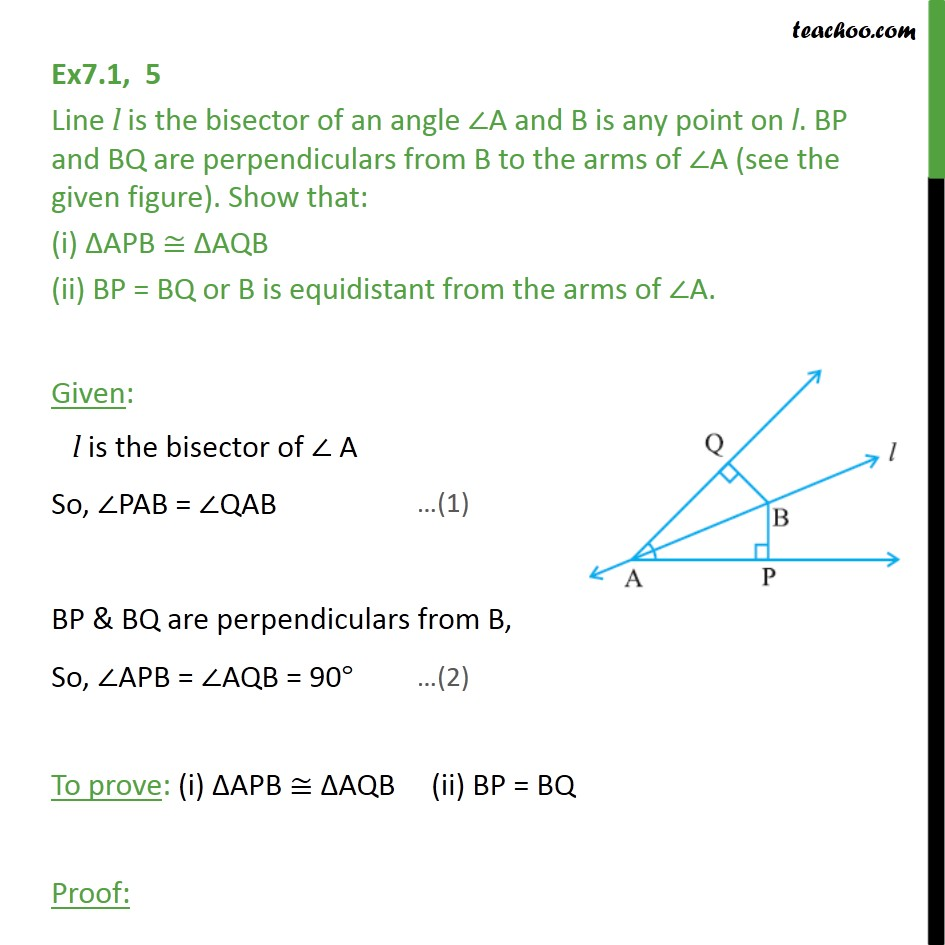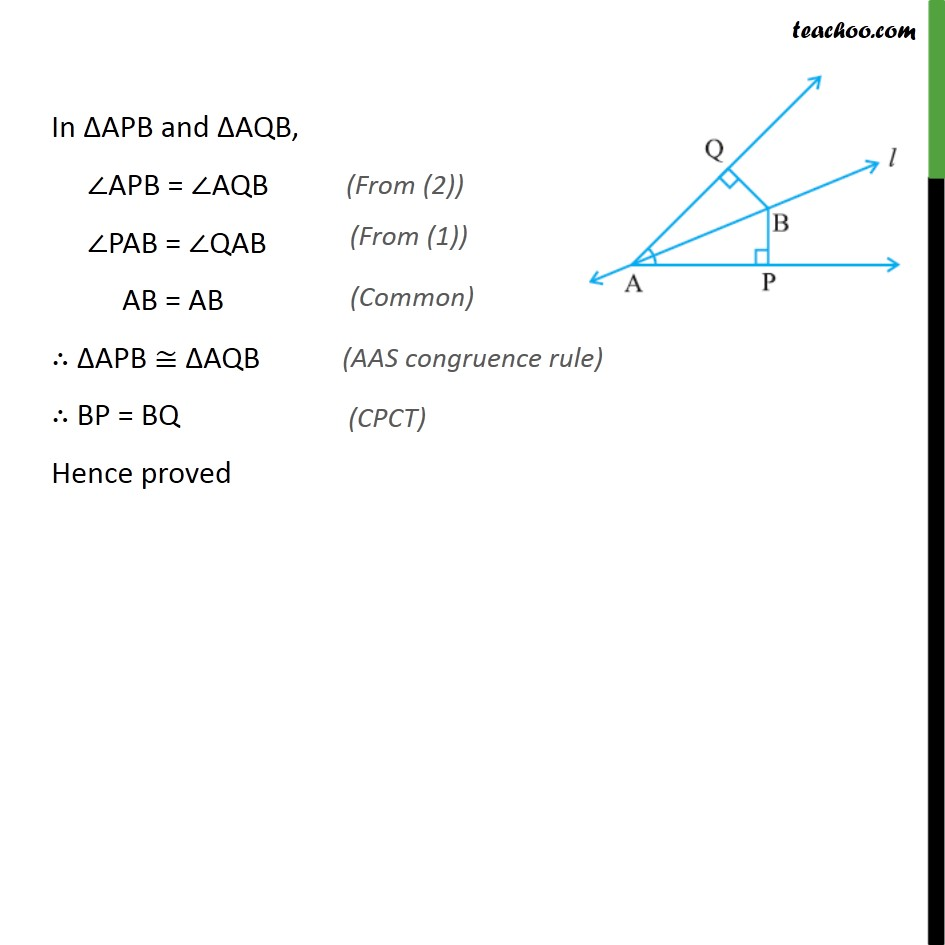Ex 7.1

Chapter 7 Class 9 Triangles
Serial order wiseLearn in your speed, with individual attention - Teachoo Maths 1-on-1 Class

### Transcript

Ex7.1, 5 Line l is the bisector of an angle A and B is any point on l. BP and BQ are perpendiculars from B to the arms of A (see the given figure). Show that: (i) APB AQB (ii) BP = BQ or B is equidistant from the arms of A. Given: l is the bisector of A So, PAB = QAB BP & BQ are perpendiculars from B, So, APB = AQB = 90 To prove: (i) APB AQB (ii) BP = BQ Proof: In APB and AQB, APB = AQB PAB = QAB AB = AB APB AQB BP = BQ Hence proved